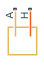# Constant Volume Chamber (TL)

Chamber with fixed volume of thermal liquid and variable number of ports

•Libraries:
Simscape / Foundation Library / Thermal Liquid / Elements

## Description

The Constant Volume Chamber (TL) block models the accumulation of mass and energy in a chamber containing a fixed volume of thermal liquid. The chamber can have between one and four inlets, labeled from A to D, through which fluid can flow. The fluid volume can exchange heat with a thermal network, such as a network representing the chamber surroundings, through the thermal port H.

The mass of the fluid in the chamber varies with density, a property that in a thermal liquid is generally a function of pressure and temperature. Fluid enters when the pressure upstream of the inlet rises above the pressure in the chamber and exits when the pressure gradient is reversed. The effect in a model is often to smooth out sudden changes in pressure, much like an electrical capacitor does with voltage.

The flow resistance between the inlet and the interior of the chamber is assumed to be negligible. The pressure in the interior is therefore equal to the pressure at the inlet. Similarly, the thermal resistance between the thermal port and the interior of the chamber is assumed to be negligible. The temperature in the interior is equal to the temperature at the thermal port.

### Mass Balance

Mass can enter and exit the chamber through ports A, B, C, and D. The volume of the chamber is fixed, but the compressibility of the fluid means that its mass can change with pressure and temperature. The rate of mass accumulation in the chamber must exactly equal the mass flow rates in through ports A, B, C, and D:

`$\left(\frac{1}{\beta }\frac{dp}{dt}-\alpha \frac{dT}{dt}\right)\rho V={\stackrel{˙}{m}}_{\text{A}}+{\stackrel{˙}{m}}_{\text{B}}+{\stackrel{˙}{m}}_{\text{C}}+{\stackrel{˙}{m}}_{\text{D}},$`

where the left-hand side is the rate of mass accumulation and:

• p is the pressure.

• T is the temperature.

• β is the isothermal bulk modulus.

• ɑ is the isobaric thermal expansion coefficient.

• $\stackrel{˙}{m}$ is the mass flow rate.

### Energy Balance

Energy can enter and exit the chamber in two ways: with fluid flow through ports A, B, C, and D, and with heat flow through port H. No work is done on or by the fluid inside the chamber. The rate of energy accumulation in the internal fluid volume must therefore equal the sum of the energy flow rates in through ports A, B, C, D, and H:

`$\left[\left(\frac{h}{\beta }-\frac{T\alpha }{\rho }\right)\frac{dp}{dt}+\left({c}_{p}-h\alpha \right)\frac{dT}{dt}\right]\rho V={\varphi }_{\text{A}}+{\varphi }_{\text{B}}+{\varphi }_{\text{C}}+{\varphi }_{\text{D}}+\text{​}{Q}_{\text{H}},$`

where the left-hand side is the rate of energy accumulation and:

• h is the enthalpy.

• ρ is the density.

• cp is the specific heat.

• V is the chamber volume.

• ϕ is the energy flow rate.

• Q is the heat flow rate.

### Momentum Balance

The pressure drop due to viscous friction between the individual ports and the interior of the chamber is assumed to be negligible. Gravity is ignored, as are other body forces. The pressure in the internal fluid volume must therefore equal the pressure at ports A, B, C, and D:

`$p={p}_{\text{A}}={p}_{\text{B}}={p}_{\text{C}}={p}_{\text{D}}.$`

### Variables

To set the priority and initial target values for the block variables prior to simulation, use the Initial Targets section in the block dialog box or Property Inspector. For more information, see Set Priority and Initial Target for Block Variables.

Nominal values provide a way to specify the expected magnitude of a variable in a model. Using system scaling based on nominal values increases the simulation robustness. Nominal values can come from different sources, one of which is the Nominal Values section in the block dialog box or Property Inspector. For more information, see Modify Nominal Values for a Block Variable.

### Assumptions and Limitations

• The chamber has a fixed volume of fluid.

• The flow resistance between the inlet and the interior of the chamber is negligible.

• The thermal resistance between the thermal port and the interior of the chamber is negligible.

• The kinetic energy of the fluid in the chamber is negligible.

## Ports

### Conserving

expand all

Thermal liquid conserving port associated with the chamber inlet.

Thermal liquid conserving port associated with the second chamber inlet.

#### Dependencies

This port is visible if you set the Number of ports parameter to `2`, `3`, or `4`.

Thermal liquid conserving port associated with the third chamber inlet.

#### Dependencies

This port is visible if you set the Number of ports parameter to `3` or `4`.

Thermal liquid conserving port associated with the fourth chamber inlet. If a chamber has four inlet ports, you can use it as a junction in a cross connection.

#### Dependencies

This port is visible only if you set the Number of ports parameter to `4`.

Thermal conserving port through which the fluid in the chamber exchanges heat with a thermal network.

## Parameters

expand all

Volume of fluid in the chamber. This volume is constant during simulation.

Number of inlet ports in the chamber. The chamber can have between one and four ports, labeled from A to D. When you modify the parameter value, the corresponding ports are exposed or hidden in the block icon.

Inlet area normal to the direction of flow.

Inlet area normal to the direction of flow.

#### Dependencies

Enabled when port B is visible, that is, when the Number of ports parameter is set to `2`, `3`, or `4`.

Inlet area normal to the direction of flow.

#### Dependencies

Enabled when port C is visible, that is, when the Number of ports parameter is set to `3` or `4`.

Inlet area normal to the direction of flow.

#### Dependencies

Enabled when port D is visible, that is, when the Number of ports parameter is set to `4`.

## Version History

Introduced in R2013b